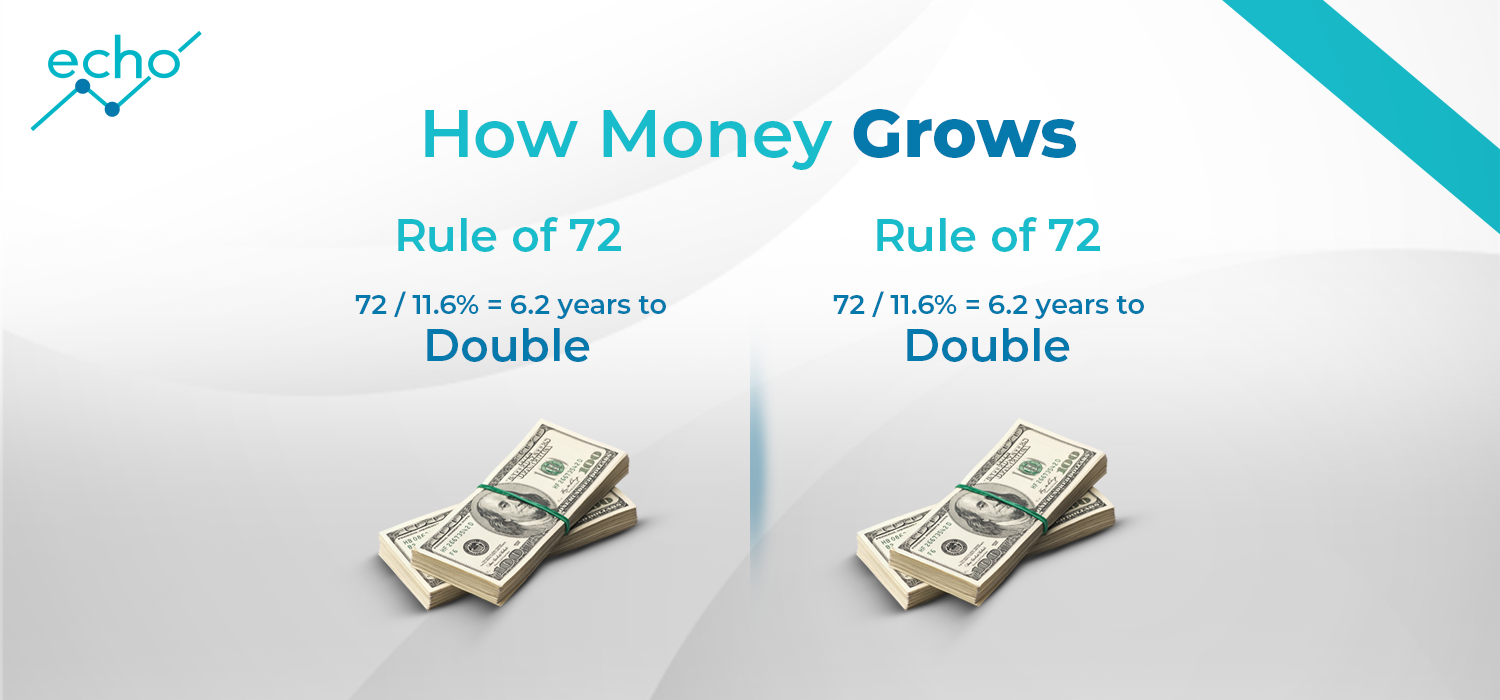## What Is the Rule of 72?

The Rule of 72 is a quick, useful formula that is popularly used to estimate the number of years required to double the invested money at a given annual rate of return

While calculators and spreadsheet programs like excel sheets have inbuilt functions to accurately calculate the precise time required to double the invested money, the Rule of 72 comes in handy for mental calculations to quickly gauge an approximate value. Alternatively, it can compute the annual rate of compounded return from an investment given how many years it will take to double the investment.